true

Kapil GargDooravaninagar, Bangalore, India - 560016# Kapil Garg## IT Professinal with 17 years of experience, tech blogger,...

Dooravaninagar, Bangalore, India - 560016.

Referral Discount: Get ₹ 500 off when you make a payment to start classes. Get started by Booking a Demo.

Details verified of Kapil Garg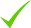IdentityEducation

Know how UrbanPro verifies Tutor details

Identity is verified based on matching the details uploaded by the Tutor with government databases.

## Overview

I have professional's degree in Computer Science and Engineering, have got 17 years of industry experience and have taken tuitions for students upto 10th class in Maths, English, Hindi and basic computer science

## Languages Spoken

Hindi Mother Tongue (Native)

English Proficient

Punjabi Basic

## Education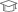Punjab Engineering College 2000

Bachelor of Engineering (B.E.)

Dooravaninagar, Bangalore, India - 560016

## Verified Info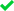ID VerifiedEducation VerifiedPhone VerifiedEmail Verified

Report this Profile

Is this listing inaccurate or duplicate? Any other problem?

Type the letters as shown below *

Please enter the letters as show below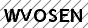Class I-V Tuition

Class LocationOnline (video chat via skype, google hangout etc)Student's HomeTutor's Home

Years of Experience in Class I-V Tuition

17

Board

State, CBSE, ICSE

CBSE Subjects taught

Mathematics, Science, English, Hindi, Computers

ICSE Subjects taught

Science, Hindi, English, Computer science, Mathematics

Taught in School or College

No

State Syllabus Subjects taught

English, Mathematics, Science, Hindi, Computer Science

## Reviews

this is test message this is test message this is test message this is test message this is test message this is test message this is test message

No Reviews yet! Be the first one to Review

## FAQs

1. Which school boards of Class 1-5 do you teach for?

State, CBSE and ICSE

2. Have you ever taught in any School or College?

No

3. Which classes do you teach?

I teach Class I-V Tuition Class.

4. Do you provide a demo class?

Yes, I provide a free demo class.

5. How many years of experience do you have?

I have been teaching for 17 years.

## Answers by Kapil Garg (1)

5(X^2+Y^2+Z^2)=4(XY+YZ+ZX). (X^2+Y^2+Z^2) is always positive since it is a sum of squares.=> left-hand side term is still positive, so by equality; the right-hand side term is also positive:: The first conclusion:- XY+YZ+ZX is always positive. Now it can be re-written as 4(X^2+Y^2+Z^2) + (X^2+Y^2+Z^2)... ...more

5(X^2+Y^2+Z^2)=4(XY+YZ+ZX).

(X^2+Y^2+Z^2) is always positive since it is a sum of squares.
=> left-hand side term is still positive, so by equality; the right-hand side term is also positive
:: The first conclusion:- XY+YZ+ZX is always positive.

Now it can be re-written as

4(X^2+Y^2+Z^2) + (X^2+Y^2+Z^2) = 4(XY+YZ+ZX)
=> 4(X^2+Y^2+Z^2) - 4(XY+YZ+ZX) + X^2+Y^2+Z^2) - 4(XY+YZ+ZX) = 0
=> 4((X^2+Y^2+Z^2) - (XY+YZ+ZX)) + X^2+Y^2+Z^2) - 4(XY+YZ+ZX) = 0

assuming (X^2+Y^2+Z^2) to be a positiver term A and
(XY+YZ+ZX) to be a positive term B
=> 4(A - B) + A = 0
=> the above relation can only be true when both positive terms A & B are 0.
=> (X^2+Y^2+Z^2) = 0
=> the above can only be true when each of X, Y & Z are 0, since squares of each will always be positive.

Thus X = Y = Z = 0 => X:Y:Z = 1:1:1

Dislike Bookmark
Class I-V Tuition

Class LocationOnline (video chat via skype, google hangout etc)Student's HomeTutor's Home

Years of Experience in Class I-V Tuition

17

Board

State, CBSE, ICSE

CBSE Subjects taught

Mathematics, Science, English, Hindi, Computers

ICSE Subjects taught

Science, Hindi, English, Computer science, Mathematics

Taught in School or College

No

State Syllabus Subjects taught

English, Mathematics, Science, Hindi, Computer Science

this is test message this is test message this is test message this is test message this is test message this is test message this is test message

No Reviews yet! Be the first one to Review

## Answers by Kapil Garg (1)

5(X^2+Y^2+Z^2)=4(XY+YZ+ZX). (X^2+Y^2+Z^2) is always positive since it is a sum of squares.=> left-hand side term is still positive, so by equality; the right-hand side term is also positive:: The first conclusion:- XY+YZ+ZX is always positive. Now it can be re-written as 4(X^2+Y^2+Z^2) + (X^2+Y^2+Z^2)... ...more

5(X^2+Y^2+Z^2)=4(XY+YZ+ZX).

(X^2+Y^2+Z^2) is always positive since it is a sum of squares.
=> left-hand side term is still positive, so by equality; the right-hand side term is also positive
:: The first conclusion:- XY+YZ+ZX is always positive.

Now it can be re-written as

4(X^2+Y^2+Z^2) + (X^2+Y^2+Z^2) = 4(XY+YZ+ZX)
=> 4(X^2+Y^2+Z^2) - 4(XY+YZ+ZX) + X^2+Y^2+Z^2) - 4(XY+YZ+ZX) = 0
=> 4((X^2+Y^2+Z^2) - (XY+YZ+ZX)) + X^2+Y^2+Z^2) - 4(XY+YZ+ZX) = 0

assuming (X^2+Y^2+Z^2) to be a positiver term A and
(XY+YZ+ZX) to be a positive term B
=> 4(A - B) + A = 0
=> the above relation can only be true when both positive terms A & B are 0.
=> (X^2+Y^2+Z^2) = 0
=> the above can only be true when each of X, Y & Z are 0, since squares of each will always be positive.

Thus X = Y = Z = 0 => X:Y:Z = 1:1:1

Dislike Bookmark

Kapil Garg describes himself as IT Professinal with 17 years of experience, tech blogger, Coach, Counsellor, Tutor. He conducts classes in Class I-V Tuition. Kapil is located in Dooravaninagar, Bangalore. Kapil takes at students Home, Regular Classes- at his Home and Online Classes- via online medium. He has 17 years of teaching experience . Kapil has completed Bachelor of Engineering (B.E.) from Punjab Engineering College in 2000. He is well versed in English, Hindi and Punjabi.

•• Want to learn from Kapil Garg?

• Contact Now
X

Recommended Profiles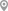Dooravaninagar, BangaloreDooravaninagar, BangaloreDooravaninagar, BangaloreDooravaninagar, BangaloreDooravaninagar, BangaloreDooravaninagar, Bangalore

X

Let us shortlist and give the best tutors and institutes.

or

Send Enquiry to Kapil Garg

Let Kapil Garg know you are interested in their class